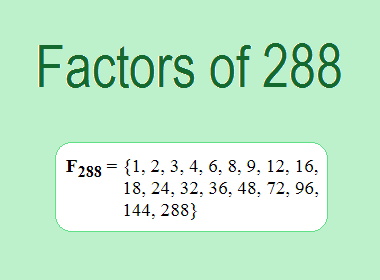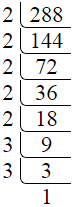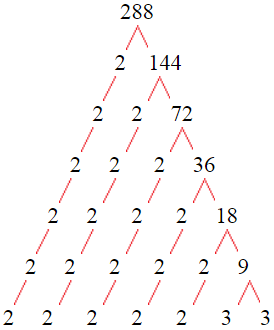# Factors of 288The factors of 288 are 1, 2, 3, 4, 6, 8, 9, 12, 16, 18, 24, 32, 36, 48, 72, 96, 144, and 288 i.e. F288 = {1, 2, 3, 4, 6, 8, 9, 12, 16, 18, 24, 32, 36, 48, 72, 96, 144, 288}. The factors of 288 are all the numbers that can divide 288 without leaving a remainder.

We can check if these numbers are factors of 288 by dividing 288 by each of them. If the result is a whole number, then the number is a factor of 288. Let's do this for each of the numbers listed above:

·        1 is a factor of 288 because 288 divided by 1 is 288.

·        2 is a factor of 288 because 288 divided by 2 is 144.

·        3 is a factor of 288 because 288 divided by 3 is 96.

·        4 is a factor of 288 because 288 divided by 4 is 72.

·        6 is a factor of 288 because 288 divided by 6 is 48.

·        8 is a factor of 288 because 288 divided by 8 is 36.

·        9 is a factor of 288 because 288 divided by 9 is 32.

·        12 is a factor of 288 because 288 divided by 12 is 24.

·        16 is a factor of 288 because 288 divided by 16 is 18.

·        18 is a factor of 288 because 288 divided by 18 is 16.

·        24 is a factor of 288 because 288 divided by 24 is 12.

·        32 is a factor of 288 because 288 divided by 32 is 9.

·        36 is a factor of 288 because 288 divided by 36 is 8.

·        48 is a factor of 288 because 288 divided by 48 is 6.

·        72 is a factor of 288 because 288 divided by 72 is 4.

·        96 is a factor of 288 because 288 divided by 96 is 3.

·        144 is a factor of 288 because 288 divided by 144 is 2.

·        288 is a factor of 288 because 288 divided by 288 is 1.

## How to Find Factors of 288?

1 and the number itself are the factors of every number. So, 1 and 288 are two factors of 288. To find the other factors of 288, we can start by dividing 288 by the numbers between 1 and 288. If we divide 288 by 2, we get a remainder of 0. Therefore, 2 is a factor of 288. If we divide 288 by 3, we get a remainder of 0. Therefore, 3 is also a factor of 288.

Next, we can check if 4 is a factor of 288. If we divide 288 by 4, we get a remainder of 0. Therefore, 4 is also a factor of 288. If we divide 288 by 5, we get a remainder of 3. Therefore, 5 is not a factor of 288. We can continue this process for all the possible factors of 288.

Through this process, we can find that the factors of 288 are 1, 2, 3, 4, 6, 8, 9, 12, 16, 18, 24, 32, 36, 48, 72, 96, 144, and 288. These are the only numbers that can divide 288 without leaving a remainder.

********************

********************

## Properties of the Factors of 288

The factors of 288 have some interesting properties. One of the properties is that the sum of the factors of 288 is equal to 819. We can see this by adding all the factors of 288 together:

1 + 2 + 3 + 4 + 6 + 8 + 9 + 12 + 16 + 18 + 24 + 32 + 36 + 48 + 72 + 96 + 144 + 288 = 819

Another property of the factors of 288 is that the prime factors of 288 are 2, and 3 only.

## Applications of the Factors of 288

The factors of 288 have several applications in mathematics. One of the applications is in finding the highest common factor (HCF) of two or more numbers. The HCF is the largest factor that two or more numbers have in common. For example, to find the HCF of 288 and 84, we need to find the factors of both numbers and identify the largest factor they have in common. The factors of 288 are 1, 2, 3, 4, 6, 8, 9, 12, 16, 18, 24, 32, 36, 48, 72, 96, 144, and 288. The factors of 84 are 1, 2, 3, 4, 6, 7, 12, 14, 21, 28, 42, and 84. The largest factor that they have in common is 12. Therefore, the HCF of 288 and 84 is 12.

Another application of the factors of 288 is in prime factorization. Prime factorization is the process of expressing a number as the product of its prime factors. The prime factors of 288 are 2, and 3 since these are the only prime numbers that can divide 288 without leaving a remainder. Therefore, we can express 288 as:

288 = 2 × 2 × 3 × 3 × 7

We can do prime factorization by division and factor tree method also. Here is the prime factorization of 288 by division method,288 = 2 × 2 × 3 × 3 × 7

Here is the prime factorization of 288 by the factor tree method,288 = 2 × 2 × 3 × 3 × 7

## Conclusion

The factors of 288 are the numbers that can divide 288 without leaving a remainder. The factors of 288 are 1, 2, 3, 4, 6, 7, 9, 12, 14, 18, 21, 28, 36, 42, 63, 84, 126, and 288. The factors of 288 have some interesting properties, such as having a sum of 819. The factors of 288 have several applications in mathematics, such as finding the highest common factor and prime factorization.